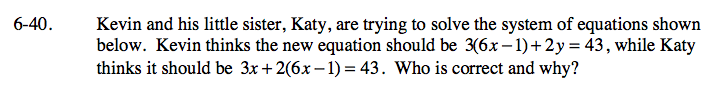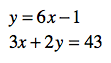### Home > CAAC > Chapter 6 > Lesson 6.2.1 > Problem6-40

6-40.

Kevin and his little sister, Katy, are trying to solve the system of equations shown below. Kevin thinks the new equation should be 3(6x − 1) + 2y = 43, while Katy thinks it should be 3x + 2(6x − 1) = 43. Who is correct and why? Homework Help ✎

y = 6x − 1

3x + 2y = 43In order to solve the system of equations 6x − 1 must be substituted in for y.

3x + 2(6x − 1) = 43

Katy is right.